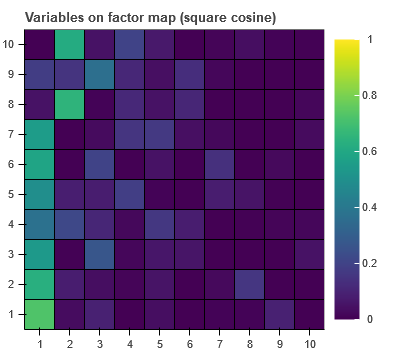# How to reverse categorical y axis?

I need to plot HeatMap (based on rect). Now, the y order is not correct since it starts from 10.You can flip the Y axis values, does this help?

``````plot=figure()
plot.y_range.flipped = True
``````

Unfortunately doesn’t work for categorical var.

unexpected attribute ‘flipped’ to FactorRange

Just guessing because I don’t know what you have to produce the plot but how about this:

``````p = figure(y_range=list(reversed(data.columns)))
``````

https://docs.bokeh.org/en/latest/docs/user_guide/categorical.html

50% success. Y axis is reversed but no data changed.

Concerning data structure:
I don’t use pandas, data are taken from numpy arrays and form CDS. Suppose the raw data could be:
x_names = [‘PC1’, ‘PC2’, ‘PC3’]
y_names = [‘var A’, ‘var B’, ‘var C’]
Z = np.array([-10,-10,-10,0,0,0,10,10,10])

@grzegorz.malinowski changing the order of the factors configured on the range as @carl suggests is the way to change the order on the axis, as described here:

https://docs.bokeh.org/en/latest/docs/user_guide/categorical.html#sorted

If you are seeing something different, then you really need to provide a complete, minimal example of your code so that is possible to see what is actually going on.

I’m a bit skeptical whether it’s a solution since we have 2D array, that must be flattened to match CDS tabular data format. But of course, let’s try. I’m providing you with the code. Y axis is in its ‘original’ position. Should be reversed, same applies to data.

``````from bokeh.io import output_notebook, output_file, show
from bokeh.models import FactorRange, ColumnDataSource, LinearColorMapper, ColorBar
from bokeh.plotting import figure
from bokeh.palettes import Viridis
output_notebook()

data = np.array([[0.7236, 0.0322, 0.0909, 0.0011, 0.0378],
[0.6307, 0.0789, 0.0363, 0.0133, 0.0544],
[0.5386, 0.0073, 0.2679, 0.0165, 0.0619],
[0.3722, 0.2164, 0.109 , 0.0209, 0.1622],
[0.4922, 0.0842, 0.0804, 0.1856, 0.0108]])

p = 5
X_names = ['1','2','3','4','5']
Y_names = ['X100m', 'Long.jump', 'Shot.put', 'High.jump', 'X400m']

x_cat_range = FactorRange(factors=X_names, range_padding=0, bounds=(0, p))
y_cat_range = FactorRange(factors=Y_names, range_padding=0, bounds=(0, p))

mapper = LinearColorMapper(Viridis, low=0, high=1)

data_m = {'X': np.tile(X_names, p),
'Y': np.repeat(Y_names, p),
'XY_values': data.flatten()}
source = ColumnDataSource(data=data_m)

p = figure(x_range=x_cat_range, y_range=y_cat_range,
frame_height=300, frame_width=300, match_aspect=True,)

p.rect(x='X', y='Y', source=source, width=1, height=1,
fill_color={'field': 'XY_values', 'transform': mapper},
line_color='black')

color_bar = ColorBar(color_mapper=mapper, location=(0, 0))